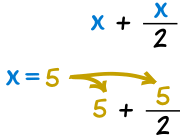Definition of

# SubstitutionPutting values where the letters are.

Example: What is x + x/2 when x=5?

Answer: Put "5" where "x" is: 5 + 5/2 = 5 + 2.5 = 7.5